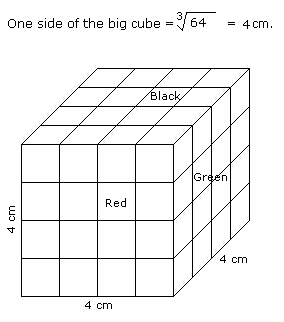# Verbal Reasoning - Cube and Cuboid - Discussion

The following questions are based on the information given below:

All the opposite faces of a big cube are coloured with red, black and green colours. After that is cut into 64 small equal cubes.

2.

How many small cubes are there whose no faces are coloured ?

 [A]. 0 [B]. 4 [C]. 8 [D]. 16

Explanation:Number of small cubes having no face coloured = (x - 2)3

= (4 - 2)3

= 8

 Chandan said: (Apr 9, 2015) Please explain it how we write (x-2)?

 Sandesh Jain said: (Jun 18, 2015) Consider volume of small cube. So each side is 2 cm (subtract 1 box on each side).